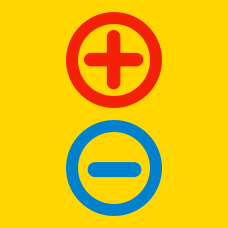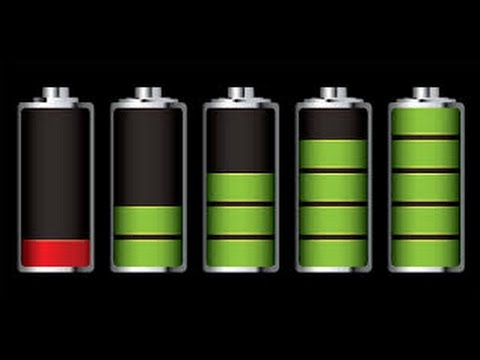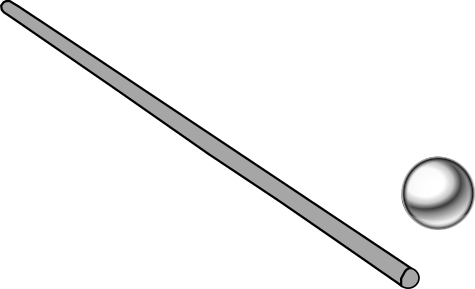Electricity and Magnetism

# Electric circuitsMost mobile phones are equipped with a Lithium-ion battery. The battery discharges when used, and needs to be charged regularly.

What change takes place in the battery as it charges?We have a metal ball and we want to find whether it is positively charged, negatively charged, or electrically neutral.

A positively charged rod is brought close to the ball, and then a negatively charged rod is brought near the ball. In both cases, the ball was attracted to the rod.

What is the charge on the ball?A resistor is an electric component that resists the flow of current. The above diagram shows two resistors connected in parallel.

What happens to the effective resistance between $A$ and $B$ if we increase the resistance of $R_1$?A resistor is an electric component that resists the flow of current. The above diagram shows two pairs of resistors: the resistors between $A$ and $B$ are connected in series, and the resistors between $C$ and $D$ are connected in parallel.

How should we connect two identical resistors to have a greater effective resistance?

How should we connect 2 identical batteries to maximize the electromotive force (EMF) between the terminals?×